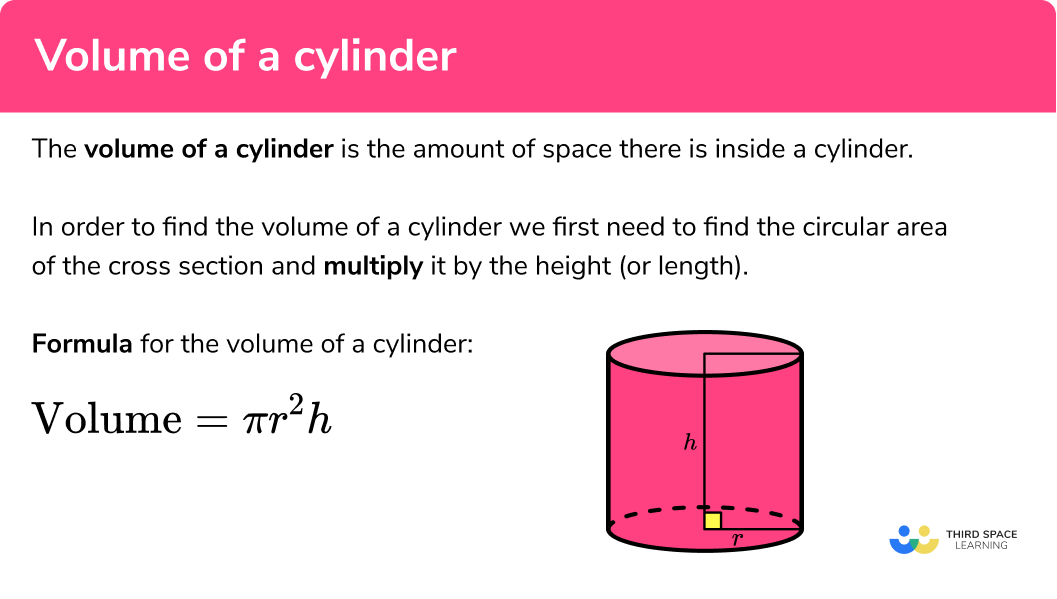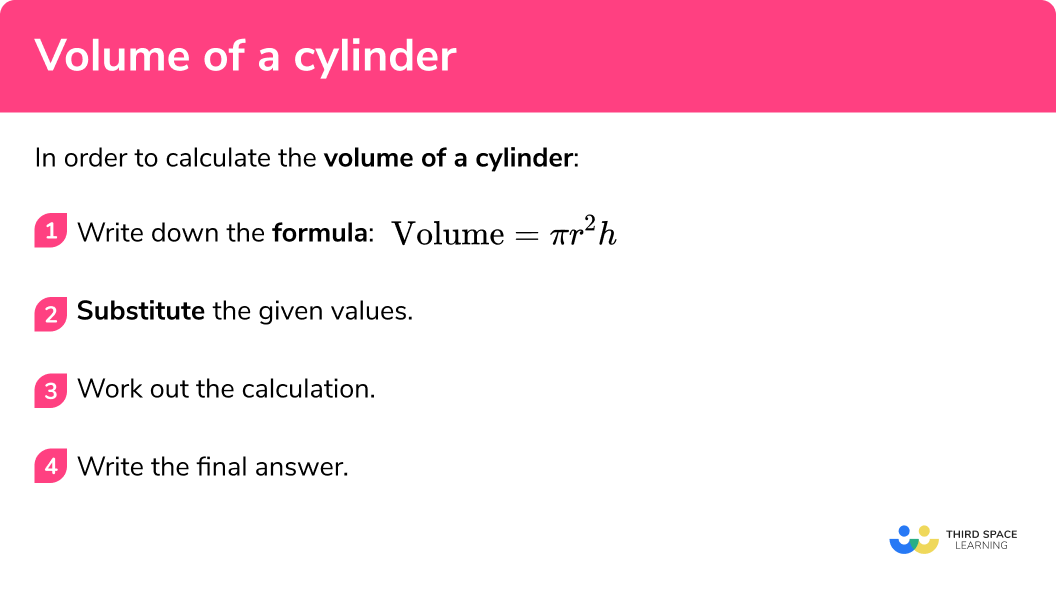# Volume Of A Cylinder

Here we will learn about the volume of a cylinder, including how to calculate the volume of a cylinder given its radius and perpendicular height.

There are also cylinder worksheets based on Edexcel, AQA and OCR exam questions, along with further guidance on where to go next if you’re still stuck.

## What is the volume of a cylinder?

The volume of a cylinder is the amount of space there is inside a cylinder.

In order to find the volume of a cylinder we first need to find the circular area of the base.

The formula for calculating the area of a circle is:

\text{Area}=\pi r^2

We then multiply the area of the circular base by the height (or length) of the cylinder.

The formula for the volume of a cylinder is:

\text{Volume}=\pi r^2 h

Where r is the radius of a cylinder and h is the perpendicular height of a cylinder.

E.g.

Find the volume of this cylinder with radius of the base 7 cm and perpendicular height 10 cm .

$\text{Volume}=\pi r^2 h\\ =\pi \times 7^2 \times 10\\ =490\pi\\ =1539.380…\\ =1539.4 \ cm^3 \ \text{(to 1 dp)}\\$

### What is the volume of a cylinder?## How to calculate the volume of a cylinder

In order to calculate the volume of a cylinder:

1. Write down the formula: \text{Volume}=\pi r^2 h
2. Substitute the given values.
3. Work out the calculation.
4. Write the final answer, including units.

### How to calculate the volume of a cylinder## Volume of a cylinder examples

### Example 1: volume of a cylinder

Find the volume of the cylinder with radius 3 cm and perpendicular height 5 cm .

1. Write down the formula.

To answer the question we need the formula for the volume of a cylinder.

\text{Volume}=\pi r^2 h

2Substitute the given values.

We need to substitute the value of the radius r and the perpendicular height h into the formula.

V=\pi \times 3^2 \times 5

3Work out the calculation.

You may need to work out volumes with of without a calculator. A calculator can be used to work out the decimal answer.

V=45\pi = 141.371…

4Write the final answer, including units.

Check what form the final answer needs to be.

You may need to leave your answer in terms of \pi or as a decimal with rounding. Here we are asked to give the answer to 1 decimal place.

V=141.371…=141.4 cm^3 \ \text{(to 1 dp)}

The volume of the cylinder is: 141.4 cm^{3} (to 1 dp)

### Example 2: volume of a cylinder

Find the volume of the cylinder with radius 4.8 cm and perpendicular height 7.9 cm .

Write down the formula.

Substitute the given values.

Work out the calculation.

Write the final answer, including units.

### Example 3: volume of a cylinder

Find the volume of the cylinder with radius 3 cm and perpendicular height 7 cm

Write down the formula.

Substitute the given values.

Work out the calculation.

Write the final answer, including units.

### Example 4: volume of a cylinder

Find the volume of the cylinder with radius 4 cm and perpendicular height 10 cm

Write down the formula.

Substitute the given values.

Work out the calculation.

Write the final answer, including units.

### Example 5: using the formula to find a length

The volume of a cylinder is 1600 cm^{3} .

Its radius is 9 cm .

Find its perpendicular height.

Write down the formula.

Substitute the given values.

Work out the calculation.

Write the final answer, including units.

### Example 6: using the formula to find a length

The volume of a cylinder is 1400 cm^{3} .

Its perpendicular height is 15 cm .

Write down the formula.

Substitute the given values.

Work out the calculation.

Write the final answer, including units.

### Common misconceptions

• Rounding

It is important to not round the answer until the end of the calculation. This will mean your final answer is accurate.

• Using the radius or the diameter

It is a common error to mix up radius and diameter. Remember the radius is half of the diameter and the diameter is double the radius.

• Correct units

For area we use square units such as cm^2 .

For volume we use cube units such as cm^3 .

Volume of a cylinder is part of our series of lessons to support revision on rounding numbers. You may find it helpful to start with the main cylinder lesson for a summary of what to expect, or use the step by step guides below for further detail on individual topics. Other lessons in this series include:

### Practice volume of a cylinder questions

1. Find the volume of a cylinder with a radius 3.2 cm and perpendicular height 9.1 cm.293 cm^3292 cm^3832 cm^3833 cm^3We are finding the volume of a cylinder so we substitute the value of r and h into the formula.

V=\pi r^2 h\\ V=\pi \times 3.2^2 \times 9.1\\ V=292.746…\\ V=293 \ cm^3 \ \text{(to 3 sf)}\\

2. Find the volume of a cylinder with a radius 5.3 cm and perpendicular height 3.8 cm .336 cm^3240 cm^3241 cm^3335 cm^3We are finding the volume of a cylinder so we substitute the value of r and h into the formula.

V=\pi r^2 h\\ V=\pi \times 5.3^2 \times 3.8\\ V=335.339…\\ V=335 \ cm^3 \ \text{(to 3 sf)}\\

3. Find the volume of a cylinder with a radius 8 cm and perpendicular height 7 cm .446\pi cm^3444\pi cm^3448\pi cm^3442\pi cm^3We are finding the volume of a cylinder so we substitute the value of r and h into the formula.

V=\pi r^2 h\\ V=\pi \times 8^2 \times 7\\ V=448\pi\\ V=448\pi \ cm^3\\

4. Find the volume of a cylinder with a radius 4 cm and perpendicular height 8 cm .402 cm^3403 cm^3128\pi cm^3128 cm^3We are finding the volume of a cylinder so we substitute the value of r and h into the formula.

V=\pi r^2 h\\ V=\pi \times 4^2 \times 8\\ V=128\pi\\ V=402 \ cm^3 (3\;\ s.f.)\\

5. The volume of a cylinder is 250 cm^3 .

Its radius is 2.9 cm .

Find its perpendicular height.

9.43 cm9.46 cm9.44 cm9.45 cmUsing the formula we substitute the value of the volume and the value of the radius and rearrange to find the radius.

V=\pi r^2 h\\ 250=\pi \times 2.9^2 \times h\\ \frac{250}{\pi \times 2.9^2}=h\\ h=9.4622…\\ h=9.46 \ cm \ \text{(to 2 dp)}\\

6. The volume of a cylinder is 800 cm^3 .

Its perpendicular height is 9.2 cm .

5.26 cm5.24 cm5.25 cm5.27 cmUsing the formula we substitute the value of the volume and the value of the perpendicular height and rearrange to find the radius.

V=\pi r^2 h\\ 800=\pi \times r^2 \times 9.2\\ 800=\pi \times r^2 \times 9.2\\ \frac{800}{\pi \times 9.2}=r^2\\ r=\sqrt{\frac{800}{\pi \times 9.2}}\\ r=5.2610…\\ r=5.26 \ cm \ \text{(to 2 dp)}\\

### Volume of a cylinder GCSE questions

1. Here is a cylinder.Calculate the volume of the cylinder.

(2 marks)

\pi \times 5.3^2 \times 4.7
For substituting the radius and the perpendicular height into the formula

(1)

414.762…=415

(1)

2. Here is a cylinder.Calculate the volume of the cylinder.

(2 marks)

\pi \times 3^2 \times 8
For substituting the radius and the perpendicular height into the formula

(1)

72\pi

(1)

3. This diagram shows a container.The container is in the shape of a cylinder.
The container is empty.

Nina has a bucket.
She is going to use the bucket to fill the container with water.

The bucket holds 8 litres of water.
How many buckets of water are needed to fill the container?

( 1 litre = 1000 cm^2 )

(4 marks)

\pi \times 25^2 \times 80
For using the formula to find the volume of the container

(1)

157 079.63…
For the correct volume

(1)

157 079.63… \div 8000
For dividing the volume of the container by the volume of the buckets with the same units

(1)

=19.63… = 20 buckets
For the correct number of buckets

(1)

## Learning checklist

You have now learned how to:

• Work out the volume of a cylinder
• Solve problems involving the volume of a cylinder

## The next lessons are

### Beyond GCSE

For GCSE we look at right circular cylinders – where the bases are parallel planes and the height is perpendicular to these bases. It is possible to have oblique cylinders.

It is also possible to have a cylinder with an ellipse as its cross-section.

## Still stuck?

Prepare your KS4 students for maths GCSEs success with Third Space Learning. Weekly online one to one GCSE maths revision lessons delivered by expert maths tutors.

Find out more about our GCSE maths tuition programme.